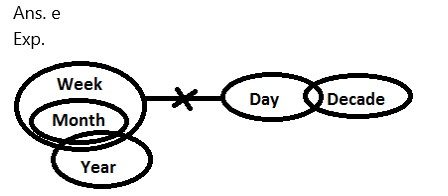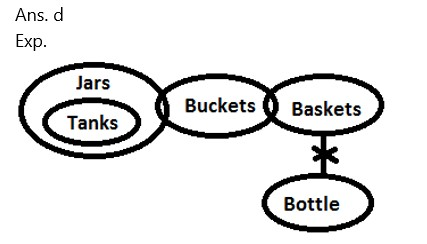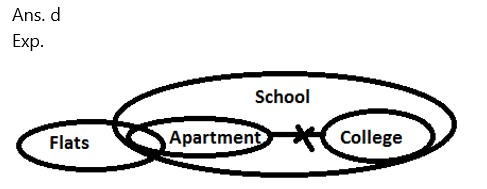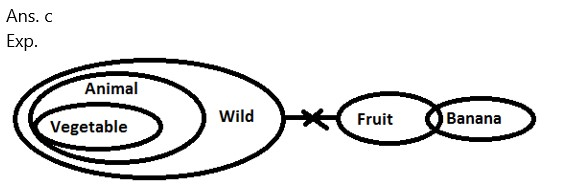# MISSION BANKING 2023 Reasoning Ability Quiz – 34

## MISSION BANKING 2023 Reasoning Ability Quiz

Aspirants have a strong possibility of scoring well in the Reasoning Ability section if they practice quality questions on a regular basis. This section takes the least amount of time if the practice is done every day in a dedicated manner. In this article, we have come up with the MISSION BANKING 2023 Reasoning Ability Quiz to help you prepare better. Candidates will be provided with a detailed solution for each question in this MISSION BANKING 2023 Reasoning Ability Quiz. This MISSION BANKING 2023 Reasoning Ability Quiz includes a variety of questions ranging in difficulty from easy to tough. This MISSION BANKING 2023 Reasoning Ability Quiz is totally FREE. This MISSION BANKING 2023 Reasoning Ability Quiz has important Reasoning Ability Questions and Answers that will help you improve your exam score. Aspirants must practice this MISSION BANKING 2023 Reasoning Ability Quiz in order to be able to answer questions quickly and efficiently in upcoming exams.

1. Statements: All months are weeks.

No week is a day.

Some month is a year.

Conclusions:

I. Some Decade is not Month.

II. Some Year are not Day.

III. Some Year are week.

(a) Only I and II

(b) None of these

(c)  Only II

(d) Only II and III

(e) Only IV1. Statements: All tanks are jars.

Some jars are Bucket.

Conclusion:

I. All Bottles being Jars is a possibility.

II. Some Buckets are not Bottle.

III. Some Bottle is not Tanks.

(a) Only IV

(b) Only I and II

(c) Only II

(d) Only III and IV

(e) None of the above1. Statements: No trees are flowers.

Some plants are flowers.

All roots are plants.

Some leaf is flower

Conclusion:

I. No Leaf are Tree.

II. Some Leaf are Plants.

III. Some Roots are Flower.

IV. Some Plant are not Tree.

(a) All except IV

(b) Only I and II

(c) Only II

(d) Only III and IV

(e) None of the above1. Statements: Some flats are apartments.

All apartments are schools.

No apartment is college.

All Colleges are School.

Conclusion:

I. Some Flats are College.

II. Some Flats are not Colleges.

III. Some Flats are School.

IV. No College are Flats.

(a) Only IV

(b) Only I and II

(c) Only II

(d) Only I and IV

(e) None of the above1. Statements: All animals are Wild.

No Wild is a fruit.

All vegetables are animals.

Some fruits are Banana.

Conclusion:

I. All Vegetable are wild.

II. Some banana are Animal.

III. Some Vegetable are not Fruit.

IV. Some Banana are not Animal.

(a) Only IV

(b) Only I and II

(c) Only II

(d) Only III and IV

(e) None of the aboveDirections (6-10): These questions are based on the following informations.

In a certain code:

‘the banner and revolt’ is coded as ‘U14  C15  M14  D15’.

‘Constitution Bench judgement’ is coded as  ‘C45  M25   M20’.

‘internal matter judiciary’ is coded as  ‘C45   S16   S14’.

1. What will be the code for ‘Judgement’?

(a) F45

(b) E45

(c) C45

(d) M14

(e) None of these

Ans. c

Exp. The given words are coded as per following pattern:

(i) First letter of the code represents the immediate previous letter of the third letter of the given word.

For example.  Bench- third letter is ‘N’=M

(ii) If the total number of letters in the word is even, then the number in the code represents the -(Total number of letters in the given word)+8. For example.  forced – total number of words = 6. 6+8=14. So code-Q14.

(iii) If the total number of letters in the given word is odd, then the number in the code represents the -(Total number of letters in the given alphabet)x5.

For example.  The- D15 (The=3, 3×5=15)

1. What will be the code for ‘Bankers Adda’?

(a) C14  M45

(b) either (c)  or (e)

(c) M35 C14

(d) C12 N45

(e) M35  C12

Ans. e

Exp. The given words are coded as per following pattern:

(i) First letter of the code represents the immediate previous letter of the third letter of the given word.

For example.  Bench- third letter is ‘N’=M

(ii) If the total number of letters in the word is even, then the number in the code represents the -(Total number of letters in the given word)+8. For example.  forced – total number of words = 6. 6+8=14. So code-Q14.

(iii) If the total number of letters in the given word is odd, then the number in the code represents the -(Total number of letters in the given alphabet)x5.

For example.  The- D15 (The=3, 3×5=15)

1. Which of the following will be the code for ‘Revenue’?

(a) U45

(b) V35

(c) V45

(d) U35

(e)  None of these

Ans. d

Exp. The given words are coded as per following pattern:

(i) First letter of the code represents the immediate previous letter of the third letter of the given word.

For example.  Bench- third letter is ‘N’=M

(ii) If the total number of letters in the word is even, then the number in the code represents the -(Total number of letters in the given word)+8. For example.  forced – total number of words = 6. 6+8=14. So code-Q14.

(iii) If the total number of letters in the given word is odd, then the number in the code represents the -(Total number of letters in the given alphabet)x5.

For example.  The- D15 (The=3, 3×5=15)

1. Which of the following can be coded as ‘B35?

(b) Context

(c) Alcohal

(d) Analyst

(e) None of these

Ans. c

Exp. The given words are coded as per following pattern:

(i) First letter of the code represents the immediate previous letter of the third letter of the given word.

For example.  Bench- third letter is ‘N’=M

(ii) If the total number of letters in the word is even, then the number in the code represents the -(Total number of letters in the given word)+8. For example.  forced – total number of words = 6. 6+8=14. So code-Q14.

(iii) If the total number of letters in the given word is odd, then the number in the code represents the -(Total number of letters in the given alphabet)x5.

For example.  The- D15 (The=3, 3×5=15)

1. What could be the code for ‘Supreme Court judges’?

(a) O35  T25  C14

(b) O35  T35  C14

(c) O35  T25  C15

(d) O25  T25  C14

(e) None of these

Ans. a

Exp. The given words are coded as per following pattern:

(i) First letter of the code represents the immediate previous letter of the third letter of the given word.

For example.  Bench- third letter is ‘N’=M

(ii) If the total number of letters in the word is even, then the number in the code represents the -(Total number of letters in the given word)+8. For example.  forced – total number of words = 6. 6+8=14. So code-Q14.

(iii) If the total number of letters in the given word is odd, then the number in the code represents the -(Total number of letters in the given alphabet)x5.

For example.  The- D15 (The=3, 3×5=15)

### 2023 Preparation Kit PDF

#### Most important PDF’s for Bank, SSC, Railway and Other Government Exam : Download PDF Now

AATMA-NIRBHAR Series- Static GK/Awareness Practice Ebook PDF Get PDF here
The Banking Awareness 500 MCQs E-book| Bilingual (Hindi + English) Get PDF here
AATMA-NIRBHAR Series- Banking Awareness Practice Ebook PDF Get PDF here
Computer Awareness Capsule 2.O Get PDF here
AATMA-NIRBHAR Series Quantitative Aptitude Topic-Wise PDF 2020 Get PDF here
Memory Based Puzzle E-book | 2016-19 Exams Covered Get PDF here
Caselet Data Interpretation 200 Questions Get PDF here
Puzzle & Seating Arrangement E-Book for BANK PO MAINS (Vol-1) Get PDF here
ARITHMETIC DATA INTERPRETATION 2.O E-book Get PDF here

3